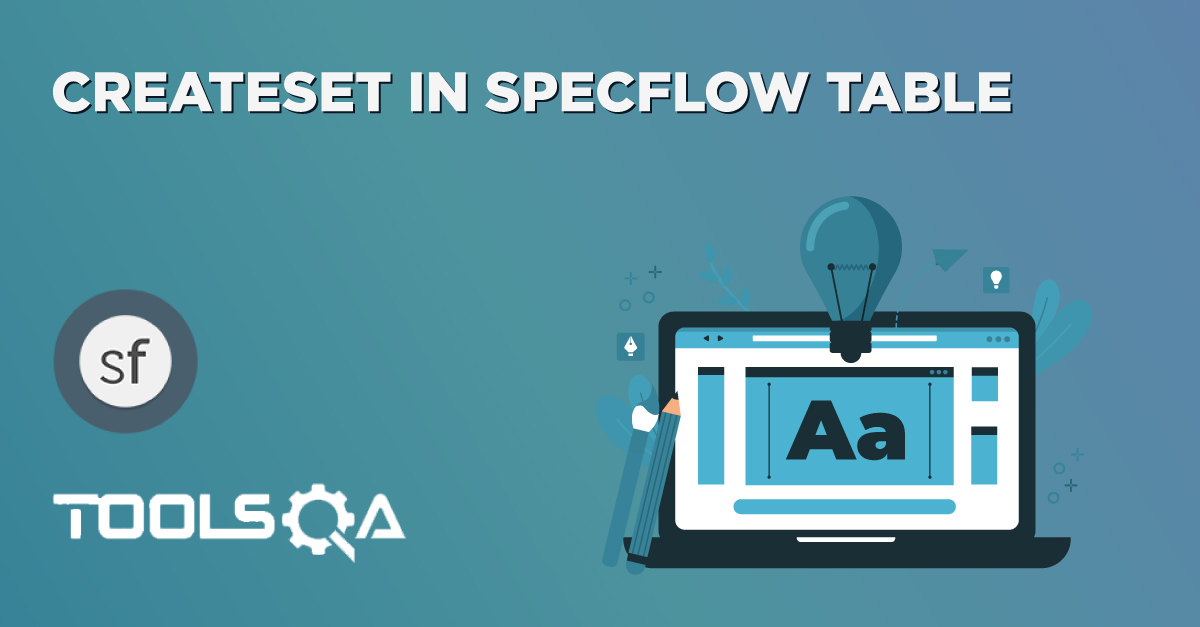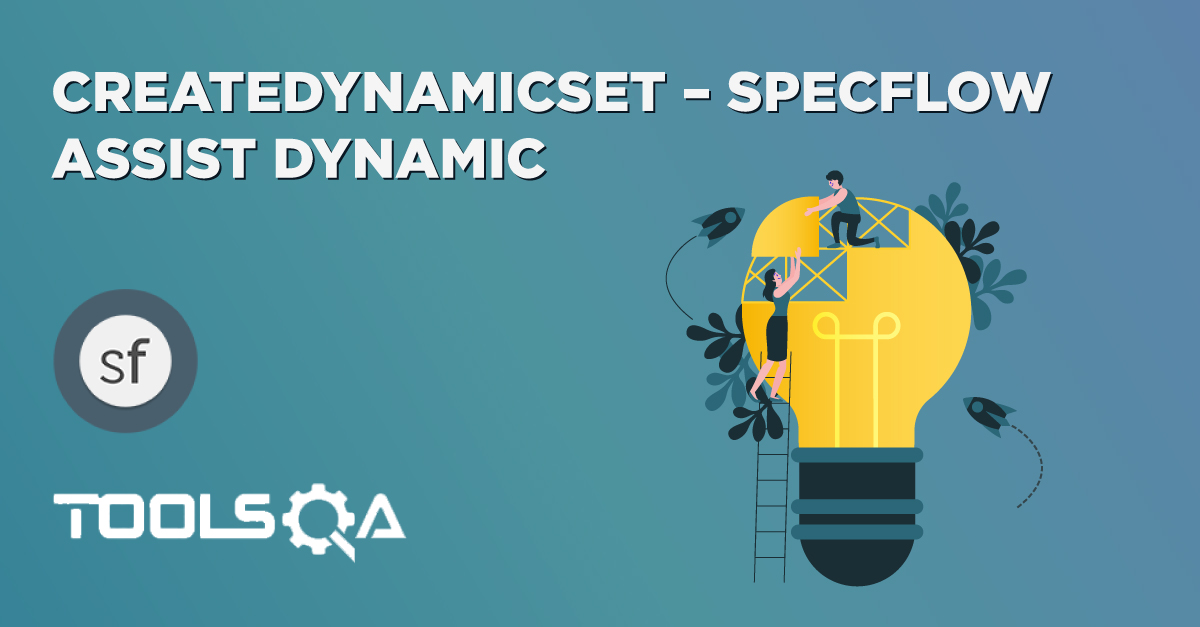## Passing Data between Steps in SpecFlow

I write a lot of BDD tests day to day using SpecFlow. I really love SpecFlow as a tool, as it allows you to write clear acceptance tests that are driven by business criteria and written in a language that the business can understand.

One of the things I like about SpecFlow is the ScenarioContext Current in SpecFlow. Sometimes you want to use data in other steps. Maybe you have generated some value types or reference types which you have to use. For instance in the Given step we initialize some input data, in the When step we use that input data to send a request to a service and then save the result, and in the Then step we execute assertions on the result.  So the question is How to store data or state during SpecFlow test steps?.

There are different ways to store data in SpecFlow steps. In this post I will introduce different ways to achieve this. Let's just take a very simple example to understand this. Once you understood it, you will be easily able to relate the same with any scenario of your Selenium Test. Below, you see a simple example where we add two numbers and verifying the total in the last Then step.

``````  Scenario: Add two numbers
Given I have entered 50 into the calculator
And I also have entered 70 into the calculator
Then the result should be 120 on the screen
``````

## Using a Private Field

The simplest solution is by using a Private field in the Step Definition class file. Simply create three different fields in the binding class, and store data in that field, which can be used in all of the steps.

Step Definition File

``````using System;
using TechTalk.SpecFlow;

namespace SpecFlowDemo.Steps
{
[Binding]
public sealed class ScenarioContextExample
{
//Three private fields to store the data across different Steps
private int firstNumber;
private int secondNumber;
private int result;

[Given("I have entered (.*) into the calculator")]
public void GivenIHaveEnteredSomethingIntoTheCalculator(int number)
{
firstNumber = number;
}

[Given(@"I also have entered (.*) into the calculator")]
public void GivenIAlsoHaveEnteredIntoTheCalculator(int number)
{
secondNumber = number;
}

{
result = firstNumber + secondNumber;
}

[Then("the result should be (.*) on the screen")]
public void ThenTheResultShouldBe(int total)
{
if (!result.Equals(total))
{
throw new Exception("Total is not correct");
}
Console.Out.WriteLine("Test Passed");
}
}
}
``````

The above solution is very simple and it requires the least amount of code, but it has one significant drawback. If we implement a feature, which has steps implemented in different binding classes, the state won't be shared among those classes. Means, let say the Then statement is in different class, in that case any of the private member would not be able to use in other class.

## Using the ScenarioContext

ScenarioContext is a static class that has a shared state during the execution of a scenario. It can be freely used as a dictionary by either storing an object of a specific type with or without specifying a string key. The ScenarioContext can be accessed by all the binding classes involved, so they can share the state.

ScenarioContext provides many different features to use, which we will also cover in detail in the following chapters. In this post we will go through the usage of ScenarioContext.Current. Using the below statements data can be passed with in the different steps of the SpecFlow test.

Set a value ScenarioContext.Current["key"] = value;

Get a value var value = ScenarioContext.Current["key"]

The only challenge in this is ScenarioContext returns an Object, it means that before using the value, it has to cast in the type in which it is meant to used. Look at the examples below:

int Type

ScenarioContext.Current["key"] = value;

var value = (int)ScenarioContext.Current["key"]

string Type

ScenarioContext.Current["key"] = value;

var value = (string)ScenarioContext.Current["key"]

Class object Type

ScenarioContext.Current["key"] = object;

var value = (objectType)ScenarioContext.Current["objectName"]

### The implementation of the same test using this approach is the following.

Step Definition File

``````using System;
using TechTalk.SpecFlow;

namespace SpecFlowDemo.Steps
{
[Binding]
public sealed class ScenarioContextExample
{
[Given("I have entered (.*) into the calculator")]
public void GivenIHaveEnteredSomethingIntoTheCalculator(int number)
{
ScenarioContext.Current["FirstNumber"] = number;
}

[Given(@"I also have entered (.*) into the calculator")]
public void GivenIAlsoHaveEnteredIntoTheCalculator(int number)
{
ScenarioContext.Current["SecondNumber"] = number;
}

{
var firstNumber = (int)ScenarioContext.Current["FirstNumber"];
var secondNumber = (int)ScenarioContext.Current["SecondNumber"];
ScenarioContext.Current["Result"] = firstNumber + secondNumber;
}

[Then("the result should be (.*) on the screen")]
public void ThenTheResultShouldBe(int total)
{
var result = ScenarioContext.Current["Result"];
if (!result.Equals(total))
{
throw new Exception("Total is not correct");
}
Console.Out.WriteLine("Test Passed");
}
}
}
``````

## ScenarioContext Current in SpecFlow

This is much nicer version of using ScenarioContext Current in SpecFlow. Does the same trick but more readable and suggested.

Set a value ScenarioContext.Current.Add(string key, object value);

Get a value var value = ScenarioContext.Current.Get`<Type>`(string Key);

### The implementation of the same test using this approach is the following.

Step Definition File

``````using System;
using System.Collections.Generic;
using System.Linq;
using System.Text;
using TechTalk.SpecFlow;

namespace SpecFlowDemo.Steps
{
[Binding]
public sealed class ScenarioContextExample
{
[Given("I have entered (.*) into the calculator")]
public void GivenIHaveEnteredSomethingIntoTheCalculator(int number)
{
}

[Given(@"I also have entered (.*) into the calculator")]
public void GivenIAlsoHaveEnteredIntoTheCalculator(int number)
{
}

{
var firstNumber = ScenarioContext.Current.Get<int>("FirstNumber");
var secondNumber = ScenarioContext.Current.Get<int>("SecondNumber");
}

[Then("the result should be (.*) on the screen")]
public void ThenTheResultShouldBe(int result)
{
var actualResult = ScenarioContext.Current.Get<int>("Result");
if (!actualResult.Equals(result))
{
throw new Exception("Total is not correct");
}
Console.Out.WriteLine("Test Passed");
}
}
}
``````

Note: This is not so difficult, but keep in mind, that the Context will be emptied for the next Scenario. You can pass data just between steps in the same Scenario.

This approach can start to get a little ugly, we also have to make sure our dictionary keys are correct in all the steps where we set or retrieve data. By default, it’s also weakly-typed – the dictionary just stores objects so we can end up with casts every time we retrieve data.

Notably, a call to Get() when the type doesn't exist e.g. Get`<BogusType>`() will result in the following exception being thrown:

System.Collections.Generic.KeyNotFoundException.ReviewersVirender Singh